## Operating-Systems

 Question 1

Consider three concurrent processes P1, P2 and P3 as shown below, which access a shared variable D that has been initialized to 100.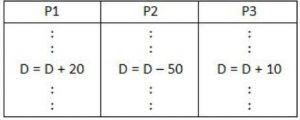The process are executed on a uniprocessor system running a time-shared operating system. If the minimum and maximum possible values of D after the three processes have completed execution are X and Y respectively, then the value of Y–X is _______.

 A 10 B 40 C 60 D 80
Operating-Systems       Process-Synchronization       GATE 2019       Video-Explanation
Question 1 Explanation:P1 executes D=D+20, D=120.
P3 executes D=D+10, D=130.
Now, P2 has D=100, executes
D = D-50 = 100-50 = 50
P2 writes D=50 final value. This is minimum.
Next,
P2 reads D=100, executes D = D-50, before that assume P1 & P3 has read D=100.
P2 makes D=50 & writes it.
P1 executes (D=100), D=D+20 & P3 executes D=D+10 gives maximum value D=130.
So, Y – X = 130 – 50 =80.
 Question 2

The following C program is executed on a Unix/Linux system:

```            #include <unistd.h>
int main ()
{
int i ;
for (i=0; i<10; i++)
if (i%2 == 0) fork ( ) ;
return 0 ;
}
```

The total number of child processes created is _____.

 A 26 B 33 C 31 D 28
Operating-Systems       System-Calls       GATE 2019       Video-Explanation
Question 2 Explanation:
Fork( ) statement is executed when ‘i’ is even. Thus the above code is equivalent to
#include
int main( )
{
int i;
fork( );
fork( );
fork( );
fork( );
fork( );
}
n – fork statements will have 2n-1 child.
Hence, n = 5 ⇒ We have 31 child processes.
 Question 3

Assume that in a certain computer, the virtual addresses are 64 bits long and the physical addresses are 48 bits long. The memory is word addressable. The page size is 8 kB and the word size is 4 bytes. The Translation Look-aside Buffer (TLB) in the address translation path has 128 valid entries. At most how many distinct virtual addresses can be translated without any TLB miss?

 A 8×220 B 4×220 C 16×210 D 256×210
Operating-Systems       Memory-Management       GATE 2019       Video-Explanation
Question 3 Explanation:
A TLB has 128 valid entries.
So, it can refer to 27 pages.
Each page size is 8 kB & word is 4 bytes.Question 4

Consider the following four processes with arrival times (in milliseconds) and their length of CPU bursts (in milliseconds) as shown below: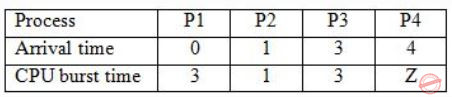These processes are run on a single processor using preemptive Shortest Remaining Time First scheduling algorithm. If the average waiting time of the processes is 1 millisecond, then the value of Z is _____.

 A 2 B 7 C 1 D 4
Operating-Systems       Process-Scheduling       GATE 2019       Video-Explanation
Question 4 Explanation:
This is the Gantt chart till time = 4 unitsAt this point P4 arrives with burst ‘Z’ & P3 is in queue with burst 3.
P1 & P2 have executed with P1 incurred delay 1unit & P2 0units.
Hence, Avg = 0+1+x/4 =1
⇒ x=3, the next delay should be 3. It would happen if assume Z=2.
It executes and completes at 6.
P3 will wait totally for 3units.
Hence, Avg=1.Z=2
 Question 5

The index node (inode) of a Unix-like file system has 12 direct, one single-indirect and one double-indirect pointers. The disk block size is 4 kB, and the disk block address is 32-bits long. The maximum possible file size is (rounded off to 1 decimal place) ______ GB.

 A 7 B 9 C 2 D 4
Operating-Systems       File system-I/O-protection       GATE 2019       Video-Explanation
Question 5 Explanation:
No. of Disk block pointers = 4kB/32bits = 1k
Max. file size
= (12 × 1k + 1k × 1k) × 4kB
≈ (1024 × 12 + 1024 × 1024) × 4 × 1024 bytes
≈ 4GB
 Question 6

Consider the following snapshot of a system running n concurrent processes. Process i is holding Xi instances of a resource R, 1 ≤ i ≤ n. Assume that all instances of R are currently in use. Further, for all i, process i can place a request for at most Yi additional instances of R while holding the Xi instances it already has. Of the n processes, there are exactly two processes p and q such that Yp = Yq = 0. Which one of the following conditions guarantees that no other process apart from p and q can complete execution?

 A Min (Xp, Xq) ≥ Min {Yk | 1 ≤ k ≤ n, k ≠ p, k ≠ q} B Xp + Xq < Max {Yk | 1 ≤ k ≤ n, k ≠ p, k ≠ q} C Min (Xp, Xq) ≤ Max {Yk | 1 ≤ k ≤ n, k ≠ p, k ≠ q} D Xp + Xq < Min {Yk | 1 ≤ k ≤ n, k ≠ p, k ≠ q}
Question 6 Explanation:
{P1, P2, …, Pn}
Pi holds Xi instances.
Pi can request additional Yi instances.
Given two process p & q such that their additional requests are zero.
Yp = Yq = 0
{Yk | 1 ≤ k ≤ n, k ≠ p, k ≠ q} means that out of ‘n’ processes, we are left with (n-2) process (except p&q), i.e., Yk indicates additional request of all the processes (n-2) except p & q.
For p & q to complete first, accordingly
Xp + Xq < Min {Yk}
Option D is correct.
There are exactly two process p and q which do not need any additional instances of resources.
So, p and q will complete their execution and will release Xp and Xq instances of resources.
Now to guarantee that no other process apart from p and q can complete execution, the no. of instances of resources available must be less than the minimum no. of instances of resources required by any other process, i.e.,
Xp + Xq < Min {Yk | 1 ≤ k ≤ n, k ≠ p, k ≠ q}.
 Question 7

Consider a process executing on an operating system that uses demand paging. The average time for a memory access in the system is M units if the corresponding memory page is available in memory and D units if the memory access causes a page fault. It has been experimentally measured that the average time taken for a memory access in the process is X units.

Which one of the following is the correct expression for the page fault rate experienced by the process?

 A (D-M)/(X-M) B (X-M)/(D-M) C (D-X)/(D-M) D (X-M)/(D-X)
Operating-Systems       Virtual Memory       GATE 2018       Video-Explanation
Question 7 Explanation:
Let ‘P’ be page fault rate then, average memory access time
X = (1 – P)M + D × P
X = M ∙ PM + DP
(X – M) = P(D – M)
⇒ P = (X – M) / (D – M)
 Question 8

Consider a system with 3 processes that share 4 instances of the same resource type. Each process can request a maximum of K instances. Resource instances can be requested and released only one at a time. The largest value of K that will always avoid deadlock is _________.

 A 2 B 3 C 4 D 5
Question 8 Explanation:
No. of process = 3
No. of resources = 4
Let’s distribute each process one less than maximum demands i.e., (k-1) resources.
So, for three processes, 3(k – 1) resources.
For deadlock avoidance provide an additional resource to any one of the process.
∴ Total resources required to avoid deadlock in any case is 3(k – 1) + 1 = 3k – 2
Now this 3k – 2 should be less than equal to available no. of resources, i.e.,
3k – 2 ≤ 4
k ≤ 2
So maximum value of k = 2
 Question 9

Consider the following solution to the producer-consumer synchronization problem. The shared buffer size is N. Three semaphores empty, full and mutex are defined with respective initial values of 0, N and 1. Semaphore empty denotes the number of available slots in the buffer, for the consumer to read from. Semaphore full denotes the number of available slots in the buffer, for the producer to write to. The placeholder variables, denoted by P, Q, R and S, in the code below can be assigned either empty or full. The valid semaphore operations are: wait() and signal().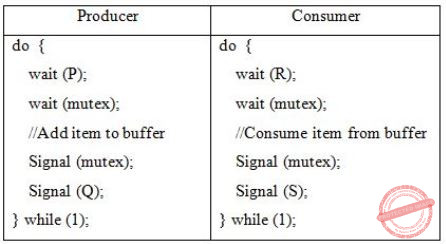Which one of the following assignments to P, Q, R and S will yield the correct solution?

 A P: full, Q: full, R: empty, S: empty B P: empty, Q: empty, R: full, S: full C P: full, Q: empty, R: empty, S: full D P: empty, Q: full, R: full, S: empty
Operating-Systems       Process-Synchronization       GATE 2018       Video-Explanation
Question 9 Explanation:
P=full, Q=empty, R=empty, S=full
Initial: mutex = 1
empty = 0
full = N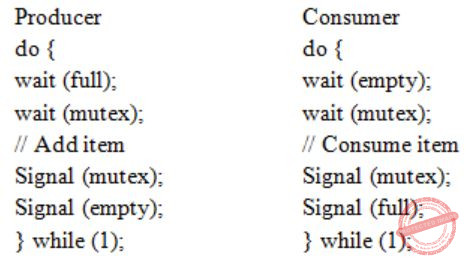Question 10

Consider a storage disk with 4 platters (numbered as 0, 1, 2 and 3), 200 cylinders (numbered as 0, 1, … , 199), and 256 sectors per track (numbered as 0, 1, … 255). The following 6 disk requests of the form [sector number, cylinder number, platter number] are received by the disk controller at the same time:

[120, 72, 2], [180, 134, 1], [60, 20, 0], [212, 86, 3], [56, 116, 2], [118, 16, 1]

Currently head is positioned at sector number 100 of cylinder 80, and is moving towards higher cylinder numbers. The average power dissipation in moving the head over 100 cylinders is 20 milliwatts and for reversing the direction of the head movement once is 15 milliwatts. Power dissipation associated with rotational latency and switching of head between different platters is negligible.

The total power consumption in milliwatts to satisfy all of the above disk requests using the Shortest Seek Time First disk scheduling algorithm is ______ .

 A 85 B 86 C 87 D 88
Operating-Systems       Disk-Scheduling       GATE 2018       Video-Explanation
Question 10 Explanation:
From the above 36 no. question we are getting
→ A storage disk – 4 platters(0, 1, 2 & 3), Cylinder – 200 (0, 1, …, 199) , 256 sector per track.
In the above question the disk requests are given in the form of 〈sector no, cylinder no, platter no〉.
In SSTF (Shortest Seek Time First) Disk Scheduling algorithm, requests having shortest seek time are executed first.
So, the seek time of every request is calculated in advance in queue and then they are scheduled according to their calculated seek time. Head is positioned at 80 and moving towards higher cylinder no.Head Movement in SSTF = (86 – 80) + (86 – 72) + (134 – 72) + (134 – 16) = 200
P1: Power dissipated by 200 movements = 0.2 * 200 = 40 mW
Power dissipated in reversing Head direction once = 15 mW
No. of times Head changes its direction = 3 P2: Power dissipated in reversing Head direction = 3 * 15 = 45 mW
Total power consumption is P1 + P2 = 85 mW
 Question 11

In a system, there are three types of resources: E, F and G. Four processes P0, P1, P2 and P3 execute concurrently. At the outset, the processes have declared their maximum resource requirements using a matrix named Max as given below. For example, Max[P2, F] is the maximum number of instances of F that P2 would require. The number of instances of the resources allocated to the various processes at any given state is given by a matrix named Allocation.

Consider a state of the system with the Allocation matrix as shown below, and in which 3 instances of E and 3 instances of F are the only resources available.From the perspective of deadlock avoidance, which one of the following is true?

 A The system is in safe state. B The system is not in safe state, but would be safe if one more instance of E were available. C The system is not in safe state, but would be safe if one more instance of F were available. D The system is not in safe state, but would be safe if one more instance of G were available.
Question 11 Explanation:
〈E, F, G〉 = 〈3, 3, 0〉Safe sequence:
〈P0, P2, P1, P3
P0: P0 can be allotted 〈3,3,0〉.
After completion Available = 〈4,3,1〉
P2: P2 can be allotted 〈0,3,0〉.
After completion: Available = 〈5,3,4〉
P1: P1 can be allotted 〈1,0,2〉.
After completion: Available = 〈6,4,6〉
P3: P3 can be allotted 〈3,4,1〉.
After completion: Available = 〈8,4,6〉
 Question 12

 A global variables but not heap. B heap but not global variables. C neither global variables nor heap. D both heap and global variables.
Operating-Systems       Threads       GATE 2017 [Set-1]       Video-Explanation
Question 12 Explanation:
Thread share all other resources of process except local data like – register, stack.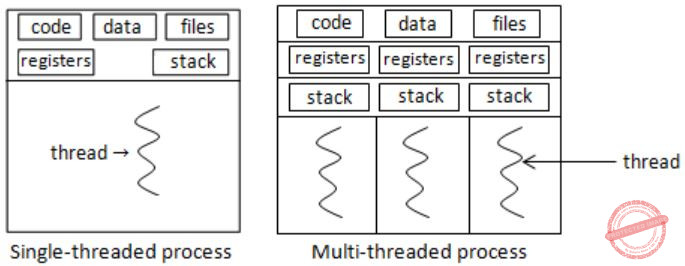Question 13

Consider the following CPU processes with arrival times (in milliseconds) and length of CPU bursts (in milliseconds) as given below: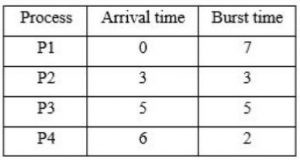If the pre-emptive shortest remaining time first scheduling algorithm is used to schedule the processes, then the average waiting time across all processes is __________ milliseconds.

 A 3 B 4 C 5 D 6
Operating-Systems       Process-Scheduling       GATE 2017 [Set-1]       Video-Explanation
Question 13 Explanation:Question 14

A multithreaded program P executes with x number of threads and used y number of locks for ensuring mutual exclusion while operating on shared memory locations. All locks in the program are non-reentrant, i.e., if a thread holds a lock l, then it cannot re-acquire lock l without releasing it. If a thread is unable to acquire a lock, it blocks until the lock becomes available. The minimum value of x and the minimum value of y together for which execution of P can result in a deadlock are:

 A x = 1, y = 2 B x = 2, y = 1 C x = 2, y = 2 D x = 1, y = 1
Operating-Systems       Process-Synchronization       GATE 2017 [Set-1]       Video-Explanation
Question 14 Explanation:
First you have to know multithreading, mutual exclusion and reentrant mutex. The reentrant mutex (recursive mutex, recursive lock) is a particular type of mutual exclusion (mutex) device that may be locked multiple times by the same process/thread, without causing a deadlock.
Here a non-reentrant process can’t own the same lock multiple times, so if the process tries to acquire an already owned lock, it will get blocked, and deadlock will happen.
From the above options x=1 (a single thread) and y=1 (a single lock) deadlock is possible when we consider the given situations in question.
 Question 15
Recall that Belady’s anomaly is that the page-fault rate may increase as the number of allocated frames increases. Now, consider the following statements:
S1: Random page replacement algorithm (where a page chosen at random is replaced) suffers from Belady’s anomaly
S2: LRU page replacement algorithm suffers from Belady’s anomaly
Which of the following is CORRECT?
 A S1 is true, S2 is true B S1 is true, S2 is false C S1 is false, S2 is true D S1 is false, S2 is false
Operating-Systems       Page-Replacement-Algorithm       GATE 2017 [Set-1]       Video-Explanation
Question 15 Explanation:
FIFO may suffer from Belady’s anomaly not always FIFO suffer from Belady’s anomaly.
Page replacement algorithm suffers from Belady’s anomaly when it is not a stack algorithm.
S1: Random page replacement algorithm is not a stack algorithm. So, S1 is true.
S2: LRU is a stack algorithm. Therefore, it doesn’t suffer from Belady’s anomaly. S2 is false.
 Question 16

Which of the following is/are shared by all the threads in a process?

I. Program counter

II. Stack

IV. Registers

 A I and II only B III only C IV only D III and IV only
Operating-Systems       Threads       GATE 2017 [Set-2]       Video-Explanation
Question 16 Explanation:
A process, in the simplest terms, is an executing program.
One or more threads run in the context of the process.
A thread is the basic unit to which the operating system allocates processor time.
A thread can execute any part of the process code, including parts currently being executed by another thread.
Each thread has its own stack, register and PC.
So here address space that is shared by all thread for a single process.
 Question 17

In a file allocation system, which of the following allocation scheme(s) can be used if no external fragmentation is allowed?

I. Contiguous

III. Indexed

 A I and III only B II only C III only D II and III only
Operating-Systems       File-Allocation-Methods       GATE 2017 [Set-2]       Video-Explanation
Question 17 Explanation:
→ Contiguous Allocation:
i) Both sequential and direct access is possible.
ii) Extremely fast.
i) Suffers from both internal and external fragmentation.
ii) Increasing file size is difficult, because it depends on the availability of contiguous memory at a particular instance.
i) Flexible in terms of size.
ii) Does not suffers from external fragmentation.
i) Allocation is slower.
ii) Does not support random or direct access.
iii) Pointers require some extra overhead.
→ Indexed allocation:
i) Support direct access, so provides fast access to the file blocks.
ii) No external fragmentation.
i) The pointer overhead for indexed allocation is greater than linked allocation.
ii) Inefficient in terms of memory utilization.
 Question 18

A system shares 9 tape drives. The current allocation and maximum requirement of tape drives for three processes are shown below:Which of the following best describe current state of the system?

Operating-Systems       deadlock       GATE 2017 [Set-2]       Video-Explanation
Question 18 Explanation:Available: (9 – (3 + 1 + 3)) = 2, P3 can be satisfied.
New available = 3 + 2 = 5
Now, P2 can be satisfied.
New available: 5 + 1 = 6
Now, P1 can be satisfied. Thus safe sequence: P3 → P2 → P1
 Question 19

Consider the set of processes with arrival time (in milliseconds), CPU burst time (in milliseconds), and priority (0 is the highest priority) shown below. None of the processes have I/O burst time.The average waiting time (in milliseconds) of all the processes using preemptive priority scheduling algorithm is __________.

 A 29 B 30 C 31 D 32
Operating-Systems       Process-Scheduling       GATE 2017 [Set-2]       Video-Explanation
Question 19 Explanation: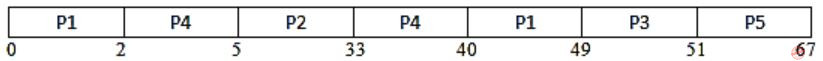Waiting Time = 0 + (33 – 5) + (40 – 2) + (49 – 12) + (51 – 9) = 145
Average waiting time: 145/5 = 29
 Question 20

Consider an arbitrary set of CPU-bound processes with unequal CPU burst lengths submitted at the same time to a computer system. Which one of the following process scheduling algorithms would minimize the average waiting time in the ready queue?

 A Shortest remaining time ﬁrst B Round-robin with time quantum less than the shortest CPU burst C Uniform random D Highest priority ﬁrst with priority proportional to CPU burst length
Operating-Systems       Process-Scheduling       GATE 2016 [Set-1]       Video-Explanation
Question 20 Explanation:
From the above question, we can get the following information.
We can consider an arbitrary set of CPU-bound processes with unequal CPU burst lengths submitted at the same time to a computer system.
We have to choose the appropriate process scheduling algorithms, which would minimize the average waiting time in the ready queue.
Waiting time is the time for which process is ready to run but not executed by CPU scheduler.
In all CPU Scheduling algorithms, shortest job first is optimal.
It gives minimum turnaround time, minimum average waiting time and high throughput and the most important thing is that shortest remaining time first is the preemptive version of shortest job first.
This scheduling algorithm may lead to starvation because if the short processes are added to the CPU scheduler continuously then the currently running process will never be able to execute as they will get pre-empted but here all the processes are arrived at same time so there will be no issue such as starvation.
SRTF would be same as SJF.
So, A is the answer. Shortest remaining time first.
 Question 21

Consider a computer system with 40-bit virtual addressing and page size of sixteen kilobytes. If the computer system has a one-level page table per process and each page table entry requires 48 bits, then the size of the per-process page table is __________ megabytes.

 A 384 MB B 385 MB C 386 MB D 387 MB
Operating-Systems       Paging       GATE 2016 [Set-1]       Video-Explanation
Question 21 Explanation:
From the above question, we got the following information.
Size of memory = 240 and Page size = 16 KB = 214
No. of pages = size of Memory / page size = 240/ 214 = 226
Size of page table = 226 * 48 / 8 bytes = 26 * 6 MB = 384 MB
 Question 22

Consider a disk queue with requests for I/O to blocks on cylinders 47, 38, 121, 191, 87, 11, 92, 10. The C-LOOK scheduling algorithm is used. The head is initially at cylinder number 63, moving towards larger cylinder numbers on its servicing pass. The cylinders are numbered from 0 to 199. The total head movement (in number of cylinders) incurred while servicing these requests is ___________.

 A 346 B 347 C 348 D 349
Operating-Systems       Disk-Scheduling       GATE 2016 [Set-1]       Video-Explanation
Question 22 Explanation:
From the question, we got the following information.
I/O to blocks on cylinders 47, 38, 121, 191, 87, 11, 92, 10.
C-LOOK scheduling algorithm is used.
Head is initially at cylinder number 63.
First start from 63
63 → 87 → 92 → 121 → 191 = 24 + 5 + 29 + 70 movements = 128
191 → 10 movements = 181
10 → 11 → 38 → 47 = 1 + 27 + 9 movements = 37
Total = 128 + 181 + 37 = 346
 Question 23

Consider a computer system with ten physical page frames. The system is provided with an access sequence (a1,a2,…,a20,a1,a2,…,a20), where each ai is a distinct virtual page number. The difference in the number of page faults between the last-in-ﬁrst-out page replacement policy and the optimal page replacement policy is ___________.

 A 1 B 2 C 3 D 4
Operating-Systems       Page-Replacement-Algorithm       GATE 2016 [Set-1]       Video-Explanation
Question 23 Explanation:
We have to calculate the difference between the last-in-first-out page replacement policy and the optimal page replacement policy.
First we can consider LIFO (Last In First Out) →
a1 to a10 will result in page faults = 10 page faults,
Then a11 will replace a10 (last in is a10), a12 replace a11 and …till a20 = 10 page faults and a20 will be top of stack and a9…a1 are remained as such.
Then a1 to a9 are already there.
So, 0 page faults from a1 to a9.
a10 will replace a20, a11 will replace a10 and so on = So 11 page faults.
So total faults will be 10+10+11 = 31.
Second Optimal Page Replacement Policy →
a1 to a10 = 10 page faults,
a11 will replace a10 because among a1 to a10, a10 will be used later, a12 will replace a11 and so on = 10 page faults.
a20 will be top of stack and a9…a1 are remained as such.
a1 to a9 = 0 page fault. a10 will replace a1 because it will not be used afterwards and so on, a10 to a19 will have 10 page faults.
So no page fault for a20.
Total = 10+ 10 +10 = 30.
So the difference between LIFO – Optimal = 1
 Question 24

Consider the following proposed solution for the critical section problem. There are n processes: P0…P(n-1). In the code, function pmax returns an integer not smaller than any of its arguments. For all i, t[i] is initialized to zero.

```Code for Pi:

do {

c[i]=1; t[i] = pmax(t,...,t[n-1])+1; c[i]=0;
for every j ≠ i in {0,...,n-1} {
while (c[j]);
while (t[j] != 0 && t[j]<=t[i]);
}
Critical Section;
t[i]=0;
Remainder Section;
} while (true);
```

Which one of the following is TRUE about the above solution?

 A At most one process can be in the critical section at any time B The bounded wait condition is satisﬁed C The progress condition is satisﬁed D It cannot cause a deadlock
Operating-Systems       Process-Synchronization       GATE 2016 [Set-1]       Video-Explanation
Question 24 Explanation:
We will check the four options one by one.
Based on the above code option B, C and D are not satisfied.
We can see that while (t[j] != 0 && t[j] <= t[i]);
because of this condition deadlock is possible when t[j] = = t[i].
Because Progress == no deadlock as no one process is able to make progress by stopping other process.
Bounded waiting is also not satisfied.
In this case both deadlock and bounded waiting to be arising from the same reason as if t[j] = = t[i] is possible then starvation is possible means infinite waiting.
Mutual exclusion is satisfied.
All other processes j started before i must have a value of t[j]) < t[i] as function pMax() return an integer not smaller than any of its arguments.
So if anyone out of the processes j have positive value will be executing in its critical section as long as the condition t[j] > 0 && t[j] <= t[i] within while will persist.
And when this j process comes out of its critical section, it sets t[j] = 0; and next process will be selected in for loop.
So, when i process reaches to its critical section none of the processes j which started earlier before process i is in its critical section.
This ensures that only one process is executing its critical section at a time.
 Question 25

In which one of the following page replacement algorithms it is possible for the page fault rate to increase even when the number of allocated frames increases?

 A LRU (Least Recently Used) B OPT (Optimal Page Replacement) C MRU (Most Recently Used) D FIFO (First In First Out)
Operating-Systems       Page-Replacement-Algorithm       GATE 2016 [Set-2]       Video-Explanation
Question 25 Explanation:
In Belady’s anomaly is the phenomenon in which increasing the number of page frames results in an increase in the number of page faults for certain memory access patterns proves that it is possible to have more page faults when increasing the number of page frames while using the First in First Out (FIFO) page replacement algorithm.
In some situations, FIFO page replacement gives more page faults when increasing the number of page frames.
 Question 26

Consider the following processes, with the arrival time and the length of the CPU burst given in milliseconds. The scheduling algorithm used is preemptive shortest remaining-time ﬁrst.The average turn around time of these processes is _________ milliseconds.

 A 8.25 B 8.26 C 8.27 D 8.28
Operating-Systems       Process-Scheduling       GATE 2016 [Set-2]       Video-Explanation
Question 26 Explanation:
Here the scheduling algorithm used is preemptive shortest remaining-time first.
To answer the question we need to design the gantt chart: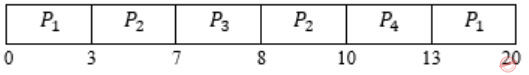In this algorithm, the processes will be scheduled on the CPU which will be having least remaining burst time.
Turnaround Time (TAT) = Completion Time (CT) – Arrival Time (AT)
TAT for P1 = 20 – 0 = 20,
TAT for P2 = 10 – 3 = 7,
TAT for P3 = 8 – 7 = 1,
TAT for P4 = 13 – 8 = 5.
Total TAT = 20 + 7 + 1 + 5 = 33 / 4 = 8.25 (Avg. TAT)
 Question 27

Consider the following two-process synchronization solution.

```Process 0                                     Process 1
---------                                     ---------
Entry: loop while (turn == 1);                Entry: loop while (turn == 0);
(critical section)                            (critical section)
Exit: turn = 1;                               Exit: turn = 0;
```

The shared variable turn is initialized to zero. Which one of the following is TRUE?

 A This is a correct two-process synchronization solution. B This solution violates mutual exclusion requirement. C This solution violates progress requirement. D This solution violates bounded wait requirement.
Operating-Systems       Process-Synchronization       GATE 2016 [Set-2]       Video-Explanation
Question 27 Explanation:
B) Mutual exclusion is satisfied because the value of the turn variable cannot be 0 and 1 at the same time.
So False.
C) Progress means if one process does not want to enter the critical section then it should not stop other process to enter the critical section.
But we can see that if process 0 will not enter the critical section then value of turn will not become 1 and process 1 will not be able to enter critical section.
So progress not satisfied. True.
D) Bounded waiting solution as there is a strict alternation.
So, False.
 Question 28

Consider a non-negative counting semaphore S. The operation P(S) decrements S, and V(S) increments S. During an execution, 20 P(S) operations and 12 V(S) operations are issued in some order. The largest initial value of S for which at least one P(S) operation will remain blocked is _________.

 A 7 B 8 C 9 D 10
Operating-Systems       Process-Synchronization       GATE 2016 [Set-2]       Video-Explanation
Question 28 Explanation:
We can assume the largest initial value of S for which at least one P(S) operation → X
P(S) operation remain in blocked state therefore it will -1.
The negative value of the counting semaphore indicates the number of processes in suspended list (or blocked).
Take any sequence of 20P and 12V operations, at least one process will always remain blocked.
So, X – 20 + 12 = -1
Here P(S) = 20 and V(S) = 12
X = 7
 Question 29

The following two functions P1 and P2 that share a variable B with an initial value of 2 execute concurrently.

```P1()
{
C = B – 1;
B = 2*C;
}

P2()
{
D = 2 * B;
B = D - 1;
}```

The number of distinct values that B can possibly take after the execution is

 A 3 B 4 C 5 D 6
Operating-Systems       Process-Synchronization       GATE 2015 [Set-1]
Question 29 Explanation:
If we execute P2 process after P1 process, then B = 3
If we execute P1 process after P2 process, then B = 4
If we did preemption between P1 & P2 processes, then B = 2 (Preemption have done from P1 to P2) or B = 3 (Preemption have done from P2 to P1). So, among 2 & 3 values, only one value will be saved in B. So, total no. of distinct values that B can possibly take after the execution is 3.
 Question 30

Consider a uniprocessor system executing three tasks T1, T2 and T3, each of which is composed of an infinite sequence of jobs (or instances) which arrive periodically at intervals of 3, 7 and 20 milliseconds, respectively. The priority of each task is the inverse of its period and the available tasks are scheduled in order of priority, with the highest priority task scheduled first. Each instance of T1, T2 and T3 requires an execution time of 1, 2 and 4 milliseconds, respectively. Given that all tasks initially arrive at the beginning of the 1st millisecond and task preemptions are allowed, the first instance of T3 completes its execution at the end of ______________ milliseconds.

 A 13 B 12 C 15 D 16
Operating-Systems       Process-Scheduling       GATE 2015 [Set-1]
Question 30 Explanation:
All the processes or tasks are available at the begining of 1st millisecond, means at t=0. So, Gantt chart will be as follows:∴ At the end of 12 milliseconds, 1st instance of T3 will complete its execution.
 Question 31

Suppose the following disk request sequence (track numbers) for a disk with 100 tracks is given: 45, 20, 90, 10, 50, 60, 80, 25, 70. Assume that the initial position of the R/W head is on track 50. The additional distance that will be traversed by the R/W head when the Shortest Seek Time First (SSTF) algorithm is used compared to the SCAN (Elevator) algorithm (assuming that SCAN algorithm moves towards 100 when it starts execution) is _________ tracks.

 A 10 B 11 C 12 D 13
Operating-Systems       Disk-Scheduling       GATE 2015 [Set-1]
Question 31 Explanation:
SCAN: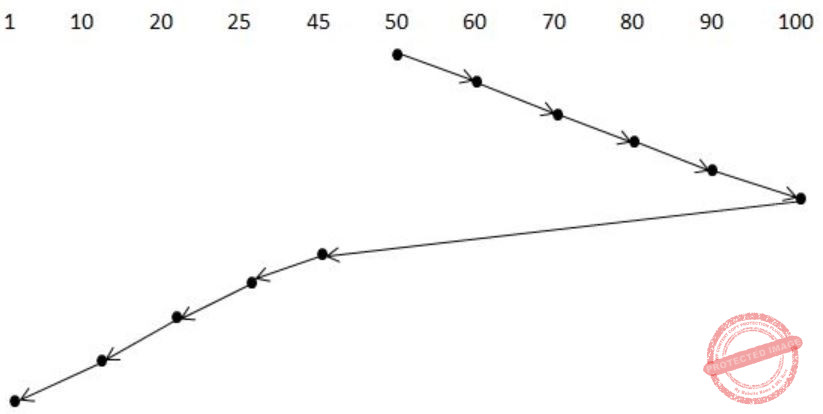= 10 + 10 + 10 + 10 + 10 + 55 + 20 + 5 + 10
= 140
SSTF: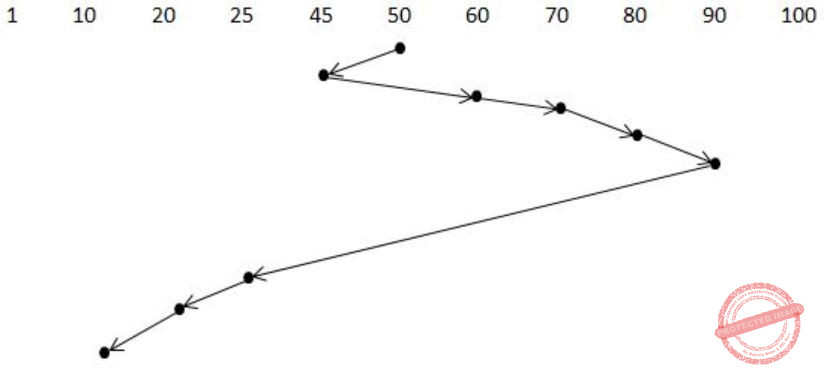= 5 + 15 + 10 + 10 + 65 + 5 + 10 + 10
= 130
= 140 – 130
= 10
 Question 32

Consider a main memory with five page frames and the following sequence of page references: 3, 8, 2, 3, 9, 1, 6, 3, 8, 9, 3, 6, 2, 1, 3. Which one of the following is true with respect to page replacement policies First-In-First Out (FIFO) and Least Recently Used (LRU)?

 A Both incur the same number of page faults B FIFO incurs 2 more page faults than LRU C LRU incurs 2 more page faults than FIFO D FIFO incurs 1 more page faults than LRU
Operating-Systems       Page-Replacement-Algorithm       GATE 2015 [Set-1]
Question 32 Explanation:∴ Number of page faults = 9∴ Number of page faults = 9
 Question 33

A system has 6 identical resources and N processes competing for them. Each process can request atmost 2 resources. Which one of the following values of N could lead to a deadlock?

 A 1 B 2 C 3 D 6
Question 33 Explanation:
Let’s assume that each process request 2 resources each. Now there are total 6 identical resources available. Give 1 resource to every processes then there will be deadlock because now each process will wait for another resource which is not available, so there will be deadlock. Since there are total 6 resources so for deadlock to be possible there should be 6 processes available. Hence, the value of N is 6.
 Question 34

A computer system implements a 40-bit virtual address, page size of 8 kilobytes, and a 128-entry translation look-aside buffer (TLB organized into 32 sets each having four ways. Assume that the TLB tag does not store any process id. The minimum length of the TLB tag in bits is _________.

 A 22 B 23 C 24 D 25
Operating-Systems       Virtual Memory       GATE 2015 [Set-2]
Question 34 Explanation:
22 bits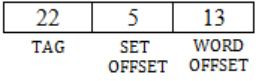Question 35

A computer system implements 8 kilobyte pages and a 32-bit physical address space. Each page table entry contains a valid bit, a dirty bit, three permission bits, and the translation. If the maximum size of the page table of a process is 24 megabytes, the length of the virtual address supported by the system is ________ bits.

 A 36 B 37 C 38 D 39
Operating-Systems       Virtual Memory       GATE 2015 [Set-2]
Question 35 Explanation:
Given page size = 8KB = 213B
PAS = 32 bit
∴ No. of frames =PA/Page size = 232 / 213 = 219
Also, it is given that each page table entry contains a valid bit, a dirty bit, 3 permission bits:
= 5 bits reserved
So one Page table entry size is
= 19+5 = 24 bits = 3 bytes
Now, Page table size = No. of entries × Entry size
24 × 220 = No. of entries × 3
No. of entries = 8 × 220 = 2 23
∴ Virtual Address size = No. of entries × Page size = 223 × 213 = 236
∴ Virtual Address Space = 36 bits
 Question 36

The maximum number of processes that can be in Ready state for a computer system with n CPUs is

 A n B n2 C 2n D Independent of n
Operating-Systems       Process-Scheduling       GATE 2015 [Set-3]
Question 36 Explanation:
Number of processes which are in running processes will be atmost n as there are n processors.
Maximum number of processes that will be in ready state is independent of number of processors.
 Question 37

Consider the following policies for preventing deadlock in a system with mutually exclusive resources.

I. Processes should acquire all their resources at the beginning of execution. If any resource is not available, all resources acquired so far are released.
II. The resources are numbered uniquely, and processes are allowed to request for resources only in increasing resource numbers.
III. The resources are numbered uniquely, and processes are allowed to request for resources only in decreasing resource numbers.
IV. The resources are numbered uniquely. A process is allowed to request only for a resource with resource number larger than its currently held resources.

Which of the above policies can be used for preventing deadlock?

 A Any one of I and III but not II or IV B Any one of I, III, and IV but not II C Any one of II and III but not I or IV D Any one of I, II, III, and IV
Question 37 Explanation:
 Question 38

For the processes listed in the following table, which of the following scheduling schemes will give the lowest average turnaround time?A First Come First Serve B Non-preemptive Shortest Job First C Shortest Remaining Time D Round Robin with Quantum value two
Operating-Systems       Process-Scheduling       GATE 2015 [Set-3]
Question 38 Explanation:FCFS:TAT for A = completion time(A) – AT(A) = 3 – 0 = 3
TAT of B = 9 – 1 = 8
TAT of C = 13 – 4 = 9
TAT of D = 15 – 6 = 9
∴ Avg. TAT = 29/4
SJF: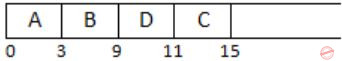TAT of A = 3 – 0 = 3
TAT of B = 9 – 1 = 8
TAT of C = 15 – 4 = 11
TAT of D = 11 – 6 = 5
∴ Avg. TAT = 27/4
SRTF:TAT of A = 3 – 0 = 3
TAT of B = 15 – 1 = 14
TAT of C = 8 – 4 = 4
TAT of D = 10 – 6 = 4
∴ Avg. TAT = 25/4
RR:TAT of A = 5 – 0 = 5
TAT of B = 15 – 1 = 14
TAT of C = 13 – 4 = 9
TAT of D = 11 – 6 = 5
∴ Avg. TAT = 33/4
∴ SRTF has lowest Avg. TAT.
 Question 39

Suppose a disk has 201 cylinders, numbered from 0 to 200. At some time the disk arm is at cylinder 100, and there is a queue of disk access requests for cylinders 30, 85, 90, 100, 105, 110, 135 and 145. If Shortest-Seek Time First (SSTF) is being used for scheduling the disk access, the request for cylinder 90 is serviced after servicing ____________ number of requests.

 A 3 B 4 C 5 D 6
Operating-Systems       Disk-Scheduling       GATE 2014 [Set-1]
Question 39 Explanation:90 is serviced after servicing three requests, i.e.,
100 → 105 → 110 → 90
 Question 40

Which one of the following is FALSE?

 A User level threads are not scheduled by the kernel. B When a user level thread is blocked, all other threads of its process are blocked. C Context switching between user level threads is faster than context switching between kernel level threads. D Kernel level threads cannot share the code segment.
Question 40 Explanation:
Kernel level threads shares the code segment.
 Question 41

An operating system uses the Banker’s algorithm for deadlock avoidance when managing the allocation of three resource types X, Y, and Z to three processes P0, P1, and P2. The table given below presents the current system state. Here, the Allocation matrix shows the current number of resources of each type allocated to each process and the Max matrix shows the maximum number of resources of each type required by each process during its execution.There are 3 units of type X, 2 units of type Y and 2 units of type Z still available. The system is currently in a safe state. Consider the following independent requests for additional resources in the current state:

```REQ1: P0 requests 0 units of X,
0 units of Y and 2 units of Z
REQ2: P1 requests 2 units of X,
0 units of Y and 0 units of Z```

Which one of the following is TRUE?

 A Only REQ1 can be permitted. B Only REQ2 can be permitted. C Both REQ1 and REQ2 can be permitted. D Neither REQ1 nor REQ2 can be permitted.
Operating-Systems       Bankers-Algorithm       GATE 2014 [Set-1]
Question 41 Explanation:
Lets take 1st case,
After allowing Req 1,Available: X=3, Y=2, Z=0
With this we can satisfy P1’s requirement. So available becomes: X=6, Y=4, Z=0.
Since Z is not available, neither P0’s nor P2’s requirement can be satisfied. So, it is an unsafe state.
Lets take 2nd case,
After allowing Req 2,Available: X=1, Y=2, Z=2
With this we can satisfy any one of P1’s or P2’s requirement. Lets first satisfy P1’s requirement. So Available now becomes:
X=6, Y=4, Z=2
Now with the availability we can satisfy P2’s requirement. So Available now becomes,
X=8, Y=5, Z=3
With this availability P0 can also be satisfied. So, hence it is in safe state.
So from above two cases Req 1 cannot be permitted but Req 2 can be permitted.
 Question 42

Consider the following set of processes that need to be scheduled on a single CPU. All the times are given in milliseconds.Using the shortest remaining time first scheduling algorithm, the average process turnaround time (in msec) is ________.

 A 7.2 B 7.3 C 7.4 D 7.5
Operating-Systems       Process-Scheduling       GATE 2014 [Set-1]
Question 42 Explanation:
Using SRTF:TAT(A) = 8-0 = 8, TAT(B)= 5-3=2, TAT(C)= 12-5=7, TAT(D)= 21-7= 14, TAT(E)=15-10=5
Average turnaround time = 8+2+7+14+5/ 5 = 7.2ms
 Question 43

Assume that there are 3 page frames which are initially empty. If the page reference string is 1, 2, 3, 4, 2, 1, 5, 3, 2, 4, 6, the number of page faults using the optimal replacement policy is__________.

 A 7 B 8 C 9 D 10
Operating-Systems       Page-Replacement-Algorithm       GATE 2014 [Set-1]
Question 43 Explanation:Total 7 faults are there.
 Question 44

A FAT (file allocation table) based file system is being used and the total overhead of each entry in the FAT is 4 bytes in size. Given a 100 × 106 bytes disk on which the file system is stored and data block size is 103 bytes, the maximum size of a file that can be stored on this disk in units of 106 bytes is ________.

 A 99.6 B 99.61 C 99.62 D 99.63
Operating-Systems       File-Allocation-Table       GATE 2014 [Set-2]
Question 44 Explanation:
Number of entry in FAT
= Total disk size/ disk block size
= 100 × 106 / 103
= 100 × 103
∴ Total overhead = 100 × 103 × 4B
= 400 × 103 B
= 0.4 × 106 B
So, Maximum size of file that can be stored in disk is,
Total disk size – Total overhead
= 100 × 106 – 0.4 × 106
= 99.6 × 106 B
 Question 45

Consider the procedure below for the Producer-Consumer problem which uses semaphores:

```semaphore n=0;
semaphore s=1;
void producer()          void consumer()
{                        {
while(true)              while(true)
{                        {
produce();               semWait(s);
semWait(s);              semWait(n);
semSignal(s);            semSignal();
semSignal(n);            consume();
}                        }
}                         }
```

Which one of the following is TRUE?

 A The producer will be able to add an item to the buffer, but the consumer can never consume it. B The consumer will remove no more than one item from the buffer. C Deadlock occurs if the consumer succeeds in acquiring semaphore s when the buffer is empty. D The starting value for the semaphore n must be 1 and not 0 for deadlock-free operation.
Operating-Systems       Process-Synchronization       GATE 2014 [Set-2]
Question 45 Explanation:
Answer is (C), because when consumer first access the semaphore ‘s’ it will down (s) and make ‘s’=0, but for semaphore(n), it has to wait for producer to make it 1 but as for producer it can’t access the critical section because the value of s=0, so semWait(s) will not work. So there will be deadlock.
 Question 46

Three processes A, B and C each execute a loop of 100 iterations. In each iteration of the loop, a process performs a single computation that requires tc CPU milliseconds and then initiates a single I/O operation that lasts for tio milliseconds. It is assumed that the computer where the processes execute has sufficient number of I/O devices and the OS of the computer assigns different I/O devices to each process. Also, the scheduling overhead of the OS is negligible. The processes have the following characteristics:

``` Process id      tc      tio
A        100 ms    500 ms
B        350 ms    500 ms
C        200 ms    500 ms```

The processes A, B, and C are started at times 0, 5 and 10 milliseconds respectively, in a pure time sharing system (round robin scheduling) that uses a time slice of 50 milliseconds. The time in milliseconds at which process C would complete its first I/O operation is ___________.

 A 1000 B 1001 C 1002 D 1003
Operating-Systems       Process-Scheduling       GATE 2014 [Set-2]
Question 46 Explanation:
Gantt chart is shown below:So ‘C’ completes its I/O at 1000 time units.
 Question 47

A computer has twenty physical page frames which contain pages numbered 101 through 120. Now a program accesses the pages numbered 1, 2, …, 100 in that order, and repeats the access sequence THRICE. Which one of the following page replacement policies experiences the same number of page faults as the optimal page replacement policy for this program?

 A Least-recently-used B First-in-first-out C Last-in-first-out D Most-recently-used
Operating-Systems       Page-Replacement-Algorithm       GATE 2014 [Set-2]
Question 47 Explanation:
The current status of 20 frames shows page numbers from 101 to 120. Implementation of optimal page replacement policy for above given page reference string would be as follows:So, there would be 300 page faults in total (each access 100 page faults). Also it is visible that every time a replacement is done for the page which is most recently referred as it will be least recently referred in future. So, for the given page reference string optimal page replacement policy is working same as most recently used policy and thus number of page faults will be same in both of them.
 Question 48

A system contains three programs and each requires three tape units for its operation. The minimum number of tape units which the system must have such that deadlocks never arise is _________.

 A 7 B 8 C 9 D 10
Question 48 Explanation:
(3*2 tape units) + 1 tape unit = 7
 Question 49

An operating system uses shortest remaining time first scheduling algorithm for pre-emptive scheduling of processes. Consider the following set of processes with their arrival times and CPU burst times (in milliseconds):The average waiting time (in milliseconds) of the processes is

 A 5.5 B 5.6 C 5.7 D 5.8
Operating-Systems       CPU-Scheduling       GATE 2014 [Set-3]
Question 49 Explanation: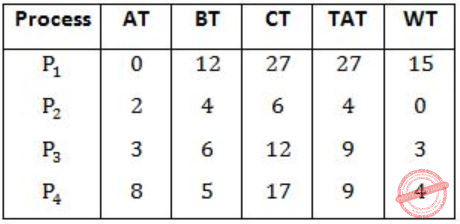WT – Waiting Time
CT – Completion Time
TAT – Turn Around Time
TAT = CT – AT < br> WT = TAT – BT
Gantt chart using Shortest remaining time first,Avg. WT = 15+0+3+4/4 = 22/4 = 5.5
 Question 50

Consider a paging hardware with a TLB. Assume that the entire page table and all the pages are in the physical memory. It takes 10 milliseconds to search the TLB and 80 milliseconds to access the physical memory. If the TLB hit ratio is 0.6, the effective memory access time (in milliseconds) is  _________.

 A 122 B 123 C 124 D 125
Operating-Systems       Memory-Management       GATE 2014 [Set-3]
Question 50 Explanation:
Tavg = TLB access time + miss ratio of TLB × memory access time + memory access time
= 10 + 0.4 × 80 + 80
= 10 + 32 + 80
= 122 ms
There are 50 questions to complete.

Register Now